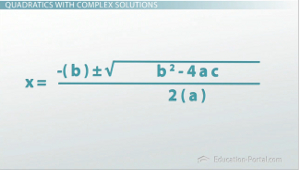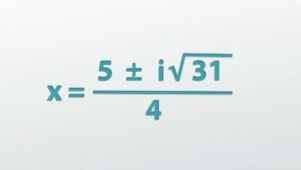# How to Solve Quadratics with Complex Numbers as the Solution

An error occurred trying to load this video.

Try refreshing the page, or contact customer support.

Coming up next: What Are the Five Main Exponent Properties?

### You're on a roll. Keep up the good work!

Replay
Your next lesson will play in 10 seconds
• 0:04 Imaginary & Complex Numbers
• 0:49 Quadratics with…
• 3:07 Polynomials with…
• 5:23 Lesson Summary

Want to watch this again later?

Timeline
Autoplay
Autoplay
Speed

#### Recommended Lessons and Courses for You

Lesson Transcript
Instructor: Luke Winspur

Luke has taught high school algebra and geometry, college calculus, and has a master's degree in education.

When you solve a quadratic equation with the quadratic formula and get a negative on the inside of the square root, what do you do? The short answer is that you use an imaginary number. For the longer, more helpful answer, check out this lesson!

## Imaginary & Complex Numbers

By allowing ourselves to imagine that square roots of negative numbers actually exist, we are able to solve a lot of real-world problems. So, what does that actually look like? How would you go about solving a problem that has an imaginary solution? That's what this lesson is all about.

You'll need to remember that imaginary numbers come about when we take the square root of a negative number, and complex numbers are when we combine a real number with an imaginary one using addition or subtraction. If this is a new concept for you, then you should check out the previous lesson that introduces these ideas, but if you already know this, we're ready to look at the example 'find the roots of y = 2x^2 - 5x + 7.'## Quadratics with Complex Solutions

When a problem asks you for the roots, it is the same thing as asking for the zeros or the x-intercepts. These are the points where y = 0, so we can substitute that value in to begin with.

Given the fact that we're doing this example in the lesson all about complex number solutions, there's a good chance that's what this problem will have. But how could we know this if this problem wasn't in this context?

It's all going to come down to the discriminant. Given a quadratic equation in standard form (y = ax^2 + bx + c), the discriminant is b^2 - 4ac. If the discriminant is positive, you'll get two real answers. If it's equal to zero, you're only going to get one real answer. But if the discriminant is negative, that's when you get two complex solutions to your problem.

This is the case because of the way the quadratic formula works. If we begin to solve the example using the quadratic formula, as shown here, you might notice that the discriminant is the part of the formula that is inside the square root. Therefore, it makes sense that if that part is negative, we'll get an imaginary number there, making our answer a complex number.

So, back to our example, if we substitute a, b and c into the formula and then begin to evaluate the expression, we'll find the discriminant will, sure enough, become negative. You'll see that we end up with the square root of -31, which is going to be an imaginary number. While this is what makes this problem new and different, the only special thing to do is to put an i in front of the square root to indicate it's imaginary, make everything else on the inside of the square root positive and then continue on just like we normally would. That's it! The i tells everyone that it is an imaginary number, but then we can move on and continue to solve the problem as normal.

As it turns out, there isn't even anything else to do with this problem; it's as simplified as it can be. Maybe if we could simplify the square root somehow, we might have a few more steps, but we can't do that, so we're good to go!## Polynomials with Complex Solutions

We can also solve polynomial problems with imaginary solutions that are bigger than quadratic equations. Take this example: Solve 0 = (x - 9)^2 * (x^2 + 9).

This isn't a quadratic equation anymore because there are two x^2s (here and here). We don't have any fancy formula for such problems like we do for quadratics, but because of the way this one is written, we can still solve it.

To unlock this lesson you must be a Study.com Member.

### Register to view this lesson

Are you a student or a teacher?

### Unlock Your Education

#### See for yourself why 30 million people use Study.com

##### Become a Study.com member and start learning now.
Back
What teachers are saying about Study.com

### Earning College Credit

Did you know… We have over 160 college courses that prepare you to earn credit by exam that is accepted by over 1,500 colleges and universities. You can test out of the first two years of college and save thousands off your degree. Anyone can earn credit-by-exam regardless of age or education level.